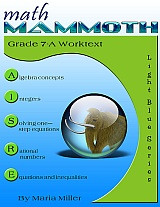Math Mammoth Grade 7-A is here!

I know it's Labor Day weekend, but...

Math Mammoth Grade 7-A is here!Please note: Only part 7-A is available for now (as of August 2014). Part B is expected to be ready sometime in early 2015.

Math Mammoth Grade 7-A is the first half of the pre-algebra curriculum from Math Mammoth. Part A worktext deals with:
• introduction to basic algebra concepts
• integers and their operations
• solving one-step equations, including with negative numbers
• operations with negative rational numbers
• solving linear equations and writing equations for word problems
• graphing linear equations and an introduction to the concept of slope

=> Order grade 7-A here (at Kagi)

Also, grade 7-A is not yet available on any of the bundle products, CDs (such as the Light Blue series CD or All Inclusive CD) nor as printed copies. You can expect it to be included in the downloadabe bundles (Light Blue series, All Inclusive, Everything Bundle) by the beginning of September and on CDs after the first week of September.

I just know there are folks "chomping at the bit" to get it so I'm sending this notice now — as early as possible — when only the download of 7-A is available.